Test: Electrical & Electronic Measurements- 5

# Test: Electrical & Electronic Measurements- 5

Test Description

## 10 Questions MCQ Test GATE Electrical Engineering (EE) 2023 Mock Test Series | Test: Electrical & Electronic Measurements- 5

Test: Electrical & Electronic Measurements- 5 for Electrical Engineering (EE) 2023 is part of GATE Electrical Engineering (EE) 2023 Mock Test Series preparation. The Test: Electrical & Electronic Measurements- 5 questions and answers have been prepared according to the Electrical Engineering (EE) exam syllabus.The Test: Electrical & Electronic Measurements- 5 MCQs are made for Electrical Engineering (EE) 2023 Exam. Find important definitions, questions, notes, meanings, examples, exercises, MCQs and online tests for Test: Electrical & Electronic Measurements- 5 below.
Solutions of Test: Electrical & Electronic Measurements- 5 questions in English are available as part of our GATE Electrical Engineering (EE) 2023 Mock Test Series for Electrical Engineering (EE) & Test: Electrical & Electronic Measurements- 5 solutions in Hindi for GATE Electrical Engineering (EE) 2023 Mock Test Series course. Download more important topics, notes, lectures and mock test series for Electrical Engineering (EE) Exam by signing up for free. Attempt Test: Electrical & Electronic Measurements- 5 | 10 questions in 30 minutes | Mock test for Electrical Engineering (EE) preparation | Free important questions MCQ to study GATE Electrical Engineering (EE) 2023 Mock Test Series for Electrical Engineering (EE) Exam | Download free PDF with solutions
 1 Crore+ students have signed up on EduRev. Have you?
Test: Electrical & Electronic Measurements- 5 - Question 1

### For the bridge shown Z1 = 200 ∠20° Ω, Z2 = 150 ∠30° Ω  and Z3 = 300 ∠-30° Ω. What is the value of Z4 so that the bridge is balanced?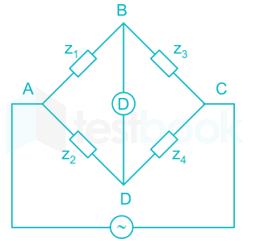Detailed Solution for Test: Electrical & Electronic Measurements- 5 - Question 1

At bridge balance condition,
Z1 Z= Z2 Z3
⇒ 200 ∠20° Z4 = 150 ∠30°    300 ∠-30°
⇒ Z4 = 225 ∠-20° Ω

*Answer can only contain numeric values
Test: Electrical & Electronic Measurements- 5 - Question 2

### A simple slide wire potentiometer is used for measurement of current in the circuit. The voltage drop across a standard resistor of 0.6Ω is balanced at 60 cm. The magnitude of current if the standard cell of 4V is balanced at 50 cm is ______ (in A)

Detailed Solution for Test: Electrical & Electronic Measurements- 5 - Question 2

Standard cell of 4 V is balanced at 50 cm.
The voltage drop across 60 cm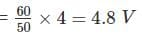The working current through the circuit is,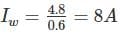Test: Electrical & Electronic Measurements- 5 - Question 3

### In the bridge circuit shown in figure when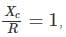then the voltmeter reads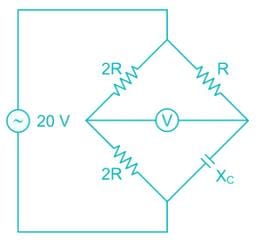Detailed Solution for Test: Electrical & Electronic Measurements- 5 - Question 3

At bridge balance condition
(2R) (Xc) = (2R) (R)
Given that,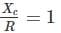at this condition, bridge is balanced
Hence the voltmeter reading is 0 V.

*Answer can only contain numeric values
Test: Electrical & Electronic Measurements- 5 - Question 4

A resistance potentiometer has a total resistance of 4 kΩ and is rated 10W. If the range of potentiometer is 0 to 200 mm. then its sensitivity is _______ (in V/mm)

Detailed Solution for Test: Electrical & Electronic Measurements- 5 - Question 4

R = 4 kΩ
P = 10 W
We know that.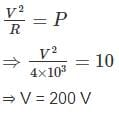The potentiometer range is 0 to 200 mm.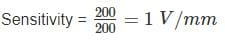Test: Electrical & Electronic Measurements- 5 - Question 5

A length of cable was tested for insulation resistance using loss of charge method. A capacitance formed by sheath of cable of 300 PF is found to have drop in voltage from 300 V to 100 V in 120 seconds. Calculate the insulation resistance of the cable in MΩ

Detailed Solution for Test: Electrical & Electronic Measurements- 5 - Question 5

Given that = C = 300 pF
V1 = 300 V
V2 = 100 V
t = 120 S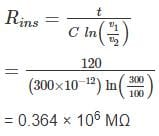*Answer can only contain numeric values
Test: Electrical & Electronic Measurements- 5 - Question 6

Calculate the value of effective resistance (in Ω) at a supply frequency of 100 Hz. (C= 2 μF)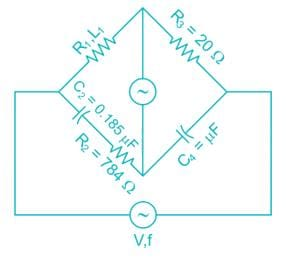Detailed Solution for Test: Electrical & Electronic Measurements- 5 - Question 6

Under balance condition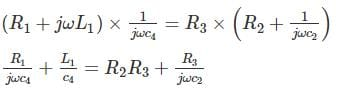Separate and equating real and imaginary part.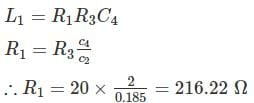*Answer can only contain numeric values
Test: Electrical & Electronic Measurements- 5 - Question 7

Find the excitation frequency (in Hz) in the Ac Bridge shown in figure under balance condition. The circuit component values are given as
R1 = 100 kΩ,
R3 = R4 = 100 kΩ,
C1 = 2 C2 = 10 nF

Detailed Solution for Test: Electrical & Electronic Measurements- 5 - Question 7

under bridge balance condition,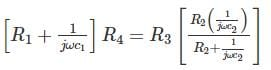Given that, R3 = R4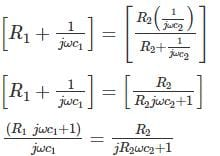(1 + jωR1C1)(1 + jωR2C2) = jωC1R2
1 + jωR1C+ jωR2C− ω2R1R2C1C= jωC1R2
By comparing real parts on both sides,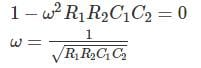By comparing imaginary parts on both sides,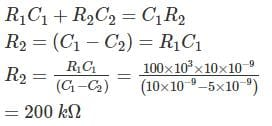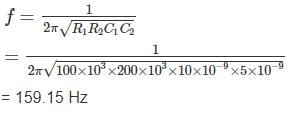Test: Electrical & Electronic Measurements- 5 - Question 8

The Schering bridge shown in figure has the following constant R1 = 1.5 kΩ, C1 = 0.4 μF, R2 = 3 kΩ and C3 = 0.4 μF at frequency 1 kHz. The dissipation factor is_______

Detailed Solution for Test: Electrical & Electronic Measurements- 5 - Question 8

Given, R1 = 1.5 kΩ
C1 = 0.4 μF
R2 = 3 kΩ
C3 = 0.4 μF
f = 1 kHz
We know that,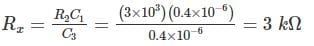and the unknown capacitance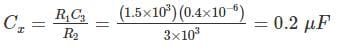Dissipation factor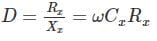= 2πfCxRx
= 2π × 1 × 103 × 0.2 × 10-6 × 3 × 103
= 3.77

*Answer can only contain numeric values
Test: Electrical & Electronic Measurements- 5 - Question 9

The AC bridge is supplied with a source of frequency 5 kHz as shown in the figure. If the bridge is balanced at R1 = 4 R2 and C3 = 20 μF, the value of unknown capacitor Cx is _____ (in μF)

Detailed Solution for Test: Electrical & Electronic Measurements- 5 - Question 9

At bridge balanced condition,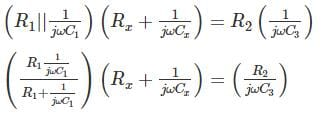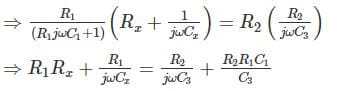By comparing imaginary part,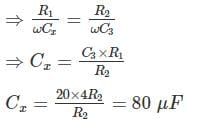Test: Electrical & Electronic Measurements- 5 - Question 10

The dc potentiometer shown in the figure has working current of 10 mA with switch S open. Let Rg + R1 = 100Ω. The galvanometer G can only detect currents greater than 10 μA. The maximum percentage error in the measurement of the unknown e.m.f. Ex as calculated from the slider position shown is closest to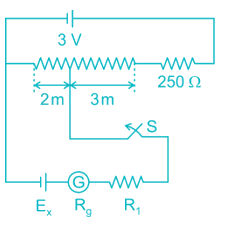Detailed Solution for Test: Electrical & Electronic Measurements- 5 - Question 10

Given that, when the switch is open.
IW = 10 mA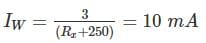⇒ Rx = 50 Ω.
Length of Rx = 5m.
Resistance across 3m = 30 Ω
Resistance across 2m = 20 Ω
Voltage across 20 Ω = 20 × 10 × 10-3 = 0.2 V
Errors in the reading are due to Rg and R1.
Error = voltage drop across Rg and R1
= Ig (Rg + R1)
= 10 × 10-6 (100) = 1 mV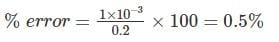## GATE Electrical Engineering (EE) 2023 Mock Test Series

22 docs|274 tests
Information about Test: Electrical & Electronic Measurements- 5 Page
In this test you can find the Exam questions for Test: Electrical & Electronic Measurements- 5 solved & explained in the simplest way possible. Besides giving Questions and answers for Test: Electrical & Electronic Measurements- 5, EduRev gives you an ample number of Online tests for practice

## GATE Electrical Engineering (EE) 2023 Mock Test Series

22 docs|274 tests(Scan QR code)